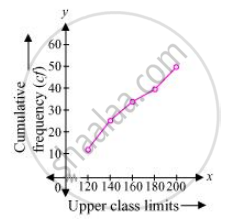Share

# The following distribution gives the daily income of 50 workers of a factory - CBSE Class 10 - Mathematics

ConceptGraphical Representation of Cumulative Frequency Distribution

#### Question

The following distribution gives the daily income of 50 workers of a factory.

 Daily income (in Rs 100 − 120 120 − 140 140 − 160 160 − 180 180 − 200 Number of workers 12 14 8 6 10

Convert the distribution above to a less than type cumulative frequency distribution, and draw its ogive.

#### Solution

The frequency distribution table of less than type is as follows

 Daily income (in Rs) (upper class limits) Cumulative frequency Less than 120 12 Less than 140 12 + 14 = 26 Less than 160 26 + 8 = 34 Less than 180 34 + 6 = 40 Less than 200 40 + 10 = 50

Taking upper class limits of class intervals on x-axis and their respective frequencies on y-axis, its ogive can be drawn as follows.Is there an error in this question or solution?

#### APPEARS IN

Solution The following distribution gives the daily income of 50 workers of a factory Concept: Graphical Representation of Cumulative Frequency Distribution.
S